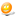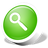HoUM

## Náhodný vtip

Koktavy vravi lekarovi: Pa pa pan do do dokk dokk tor coo jjje to ppp ro ssta ta? Doktor sa neho pozrie a vravi: Prostata je taka choroba, ked niekto moci tak, ako vy rozpravate.

viac vtipov# Stats/Math JokesSearch for your joke
Jokes found: 8543

|Theorem : All numbers are equal to zero.Proof: Suppose that a=b. Thena = ba^2 = aba^2 - b^2 = ab - b^2(a + b)(a - b) = b(a - b)a + b = ba = 0Furthermore if a + b = b, and a = b, then b + b = b, and 2b = b, which mean that 2 = 1.

|Theorem: 3=4Proof:Suppose:a + b = cThis can also be written as:4a - 3a + 4b - 3b = 4c - 3cAfter reorganizing:4a + 4b - 4c = 3a + 3b - 3cTake the constants out of the brackets:4 * (a+b-c) = 3 * (a+b-c)Remove the same term left and right:4 = 3

|Theorem: All positive integers are equal.Proof: Sufficient to show that for any two positive integers, A and B, A = B.Further, it is sufficient to show that for all N > 0, if A and B (positive integers) satisfy (MAX(A, B) = N) then A = B.Proceed by induction.If N = 1, then A and B, being positive integers, must both be 1. So A = B.Assume that the theorem is true for some value k. Take A and B with MAX(A, B) = k+1. Then MAX((A-1), (B-1)) = k. And hence (A-1) = (B-1). Consequently, A = B.

|Theorem: 1\$ = 1c.Proof:And another that gives you a sense of money disappearing.1\$ = 100c= (10c)^2= (0.1\$)^2= 0.01\$= 1cHere \$ means dollars and c means cents. This one is scary in that I have seen PhD's in math who were unable to see what was wrong with this one. Actually I am crossposting this to sci.physics because I think that the latter makes a very nice introduction to the importance of keeping track of your dimensions.

|Theorem: 1\$ = 10 centProof:We know that \$1 = 100 centsDivide both sides by 100\$ 1/100 = 100/100 cents=> \$ 1/100 = 1 centTake square root both side=> squr(\$1/100) = squr (1 cent)=> \$ 1/10 = 1 cent Multiply both side by 10=> \$1 = 10 cent

|Theorem: n=n+1Proof:(n+1)^2 = n^2 + 2*n + 1Bring 2n+1 to the left:(n+1)^2 - (2n+1) = n^2Substract n(2n+1) from both sides and factoring, we have:(n+1)^2 - (n+1)(2n+1) = n^2 - n(2n+1)Adding 1/4(2n+1)^2 to both sides yields:(n+1)^2 - (n+1)(2n+1) + 1/4(2n+1)^2 = n^2 - n(2n+1) + 1/4(2n+1)^2This may be written:[ (n+1) - 1/2(2n+1) ]^2 = [ n - 1/2(2n+1) ]^2Taking the square roots of both sides:(n+1) - 1/2(2n+1) = n - 1/2(2n+1)Add 1/2(2n+1) to both sides:n+1 = n

|Theorem: e=1Proof:2*e = f2^(2*pi*i)e^(2*pi*i) = f^(2*pi*i)e^(2*pi*i) = 1Therefore:2^(2*pi*i) = f^(2*pi*i)2=fThus:e=1

|Theorem: 1 = 1/2:Proof:We can re-write the infinite series 1/(1*3) + 1/(3*5) + 1/(5*7) + 1/(7*9)+...as 1/2((1/1 - 1/3) + (1/3 - 1/5) + (1/5 - 1/7) + (1/7 - 1/9) + ... ).All terms after 1/1 cancel, so that the sum is 1/2.We can also re-write the series as (1/1 - 2/3) + (2/3 - 3/5) + (3/5 - 4/7)+ (4/7 - 5/9) + ...All terms after 1/1 cancel, so that the sum is 1.Thus 1/2 = 1.

|Prove that the crocodile is longer than it is wide.Lemma 1. The crocodile is longer than it is green: Let's look at the crocodile. It is long on the top and on the bottom, but it is green only on the top. Therefore, the crocodile is longer than it is green.Lemma 2. The crocodile is greener than it is wide: Let's look at the crocodile. It is green along its length and width, but it is wide only along its width. Therefore, the crocodile is greener than it is wide.From Lemma 1 and Lemma 2 we conclude that the crocodile is longer than it is wide.

|Theorem: log(-1) = 0Proof:a. log[(-1)^2] = 2 * log(-1)On the other hand:b. log[(-1)^2] = log(1) = 0Combining a) and b) gives:2* log(-1) = 0Divide both sides by 2:log(-1) = 0

|Theorem: 4 = 5Proof:-20 = -2016 - 36 = 25 - 454^2 - 9*4 = 5^2 - 9*54^2 - 9*4 + 81/4 = 5^2 - 9*5 + 81/4(4 - 9/2)^2 = (5 - 9/2)^24 - 9/2 = 5 - 9/24 = 5

|Theorem: All numbers are equal.Proof: Choose arbitrary a and b, and let t = a + b. Thena + b = t(a + b)(a - b) = t(a - b)a^2 - b^2 = ta - tba^2 - ta = b^2 - tba^2 - ta + (t^2)/4 = b^2 - tb + (t^2)/4(a - t/2)^2 = (b - t/2)^2a - t/2 = b - t/2a = bSo all numbers are the same, and math is pointless.

|Theorem: 1 = -1Proof:1 = sqrt(1) = sqrt(-1 * -1) = sqrt(-1) * sqrt(-1) = 1^ = -1Also one can disprove the axiom that things equal to the same thing are equal to each other.1 = sqrt(1)-1 = sqrt(1)Therefore 1 = -1As an alternative method for solving:Theorem: 1 = -1Proof:x=1x^2=xx^2-1=x-1(x+1)(x-1)=(x-1)(x+1)=(x-1)/(x-1)x+1=1x=00=1=> 0/0=1/1=1